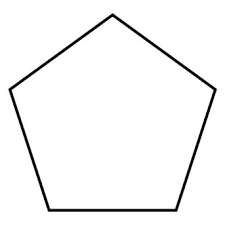## How to Calculate and Solve for the Length of a Side and Perimeter of a Pentagon | Nickzom CalculatorThe image above is a pentagon.

To compute the perimeter of a pentagon, one essential parameter is needed and this parameter is length of side (a).

The formula for calculating the perimeter of a pentagon:

P = 5a

Where;
P = Perimeter of the pentagon
a = length of side

Let’s solve an example;
Find the perimeter of a pentagon when the length of side (a) is 25 cm.

This implies that;
a = length of side = 25 cm

P = 5a
P = 5 x 25
P = 125

Therefore, the perimeter of the pentagon is 125 cm.

Calculating the length of side using the perimeter of the pentagon.

a = P / 5

Where;
a = Length of side
P = Perimeter of the pentagon

Let’s solve an example;
Given that the area of the pentagon is 250 cm. Find the length of side?

This implies that;
P = Perimeter of the pentagon = 250 cm

a = P / 5
a = 250 / 5
a = 50

Therefore, the length of side (a) is 50 cm.

## How to Calculate and Solve for the Length and Area of a Pentagon | Nickzom CalculatorThe image above is a pentagon.

To compute the area of a pentagon, one essential parameter is needed and this parameter is length of side (a).

The formula for calculating the area of a pentagon:

A = a2(5(5 + 2√5) / 4

Where;
A = Area of the pentagon
a = length of side

Let’s solve an example;
Find the area of the pentagon when the length of side is 30 cm.

This implies that;
a = length of side = 30 cm

A = a2(5(5 + 2√5) / 4
A = 302(5(5 + 4.47) / 4
A = 900√(5(9.47) / 4
A = 900√47.36 / 4
A = 900√11.84
A = 900 x 3.44
A = 3096

Therefore, the area of the pentagon is 3096 cm2.

Calculating the length of side using the area of the pentagon.

a = √(4A / √5(5 + 2√5))

Where;
a = length of side
A = Area of the pentagon

Let’s solve an example;
Find the length of side with an area of 150 cm2.

This implies that;
A = Area of the pentagon = 150 cm2

a = √(4A / √5(5 + 2√5))
a = √(4 x 150 / √5(5 + 1.148))
a = √(600 / √5(6.148))
a = √(600 / √30.74)
a = √(600 / 5.54)
a = √108.30
a = 10.41

Therefore, the length of side is 10.41 cm.